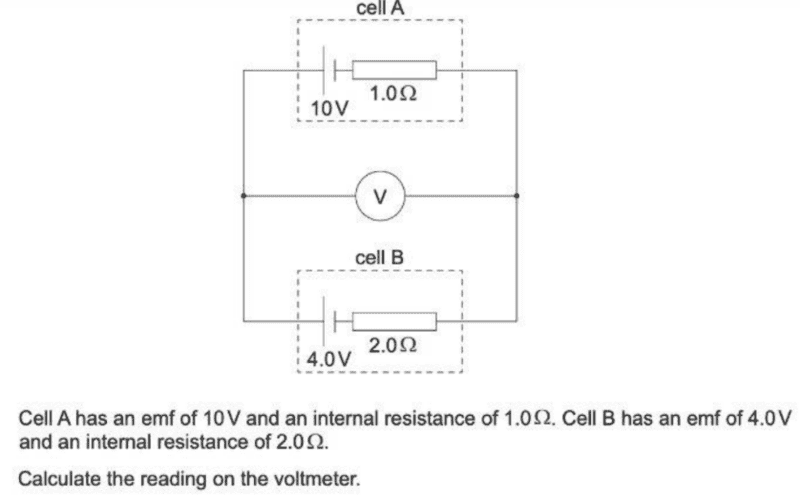# Calculate the voltmeter reading for this circuit with 2 sources and 2 resistors

• songoku

#### songoku

Homework Statement
Relevant Equations
V = I.RI find the current first, which is 2 A. Then the voltmeter reading would be 10 V - 1 x 2 = 8 V.

But the answer is 12 V.
I don't understand why it should be 10 + 1 x 2 = 12 V

Thanks

I agree with your answer. It obviously cannot be more than 10V.

•songoku and MatinSAR
Thank you very much haruspex

Yes, I agree, 8V is correct.

•songoku